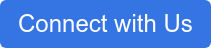Tech

# How much does it cost to charge an EV?How much will you pay to charge your EV? The answer to this question takes some calculations.

Switching from gas-powered to electric vehicles is an exciting step toward a greener world. As energy companies race to keep up with the growing demand for charging stations and affordable electric cars, customers are wondering: How much does it cost to charge an EV?

The answer to this question takes some calculations, so we’ve broken down the figures so you know how to budget your charging costs each month.

## Electricity costs

To kick things off, let’s look at what the average American would spend per hour charging their EV. The best way to calculate this on your own is by dividing your electric bill by the total number of Kilowatts used per hour (kWh). Then take how many kWh your vehicle runs on and multiply that by how much you typically pay in electricity per hour.

According to the Energy Information Administration (EIA), the average home in the U.S. in 2021 was charged almost \$0.14 per kWh. Taking this number for our example, newer EVs can hold up to 100 kWh. This means it would cost you \$14 to charge your EV to full capacity if you “fueled” up at home.

Another way to consider how much you will likely pay to charge your EV is by breaking it down by the mathematical statement: The electricity cost per day is equal to the energy consumption, “E”, in kWh per day multiplied by the energy cost of 1 kWh in cents per kWh divided by 100 cents per dollar.

Cost(\$/day) = E(kWh/day) × Cost(cent/kWh) / 100(cent/\$).

### Comparing EV costs with gas costs

In 2021 so far, the average cost of gas hovers around \$3.16 according to a study done by AAA. Using our 100 kWh EV, it can drive 300 miles before having to refuel. Of course, this can vary depending on whether you’re driving on a hilly road or you’re stuck in traffic. However, on a straight highway, this electric car can drive up to 300 miles.

Let’s compare that to how much a typical sedan would cost to drive just as far.

The average sedan can hold between 11 and 18 gallons of gas at a time and the average miles per gallon (MPG) sits between 31 and 39. If the cost of gas is \$3.16 a gallon, it will cost close to \$35 to fill up an 11-gallon tank and up to \$56 to fill up an 18-gallon tank. Driving 300 miles in the average sedan could cost between \$28 and \$25.

Therefore, the cost of filling up your tank and driving just as far as a high-powered EV could cost you twice as much at the gas pump as it does at an outlet.

## Save more, go further

Want to know how much you can save by investing in an electric car?

Contact us at Webasto and read our blog for more EV tips and trends.